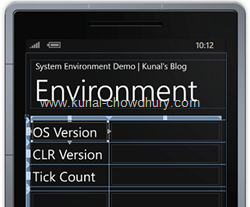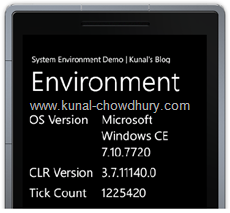# Detecting System Information of WP7 DeviceSometime we want to show the System Information to the user, whether it is an Application or a simple Game. We need to show those system specific information to the Windows Phone 7 user by using an System page. So, in such case, how will you fetch those information and show them to the user?

Under the System namespace, we have a class called “Environment” which has different properties to return information about the Windows Phone 7 device. The exposed APIs are as mentioned below. Read the comments section to know more about those here:

` `
`namespace System`
`{`
`    // Summary:`
`    //     Provides information about, and means to manipulate, the current environment`
`    //     and platform. This class cannot be inherited.`
`    public static class Environment`
`    {`
`        // Summary:`
`        //     When called by trusted applications, gets the fully qualified path of the`
`        //     current working directory.`
`        public static string CurrentDirectory { get; set; }`
` `
`        // Summary:`
`        //     Gets a value indicating whether the common language runtime is shutting down`
`        //     or the current application domain is unloading.`
`        public static bool HasShutdownStarted { get; }`
` `
`        // Summary:`
`        //     Gets the newline string defined for this environment.`
`        public static string NewLine { get; }`
` `
`        // Summary:`
`        //     Gets an System.OperatingSystem object that contains the current platform`
`        //     identifier and version number.`
`        public static OperatingSystem OSVersion { get; }`
` `
`        // Summary:`
`        //     Gets the number of processors on the current machine.`
`        public static int ProcessorCount { get; }`
` `
`        // Summary:`
`        //     Gets the number of milliseconds elapsed since the system started.`
`        public static int TickCount { get; }`
` `
`        // Summary:`
`        //     Gets a System.Version object that describes the major, minor, build, and`
`        //     revision numbers of the common language runtime.`
`        public static Version Version { get; }`
` `
`        // Summary:`
`        //     Gets the path to the system special folder identified by the specified         //     enumeration.`
`        [SecurityCritical]`
`        public static string GetFolderPath(Environment.SpecialFolder folder);`
` `
`        // Summary:`
`        //     When it is called by trusted applications, specifies enumerated constants`
`        //     used to retrieve directory paths to system special folders.`
`        public enum SpecialFolder`
`        {`
`            // Summary: The directory that contains the user's program groups.`
`            Programs = 2,`
` `
`            // Summary: The directory that serves as a common repository for documents.`
`            Personal = 5,`
` `
`            // Summary: The directory that corresponds to the user's Startup program group.`
`            Startup = 7,`
` `
`            // Summary: The directory that contains the Start menu items.`
`            StartMenu = 11,`
` `
`            // Summary: The directory that serves as a common repository for the user's             // favorite items.`
`            Favorites = 22,`
` `
`            // Summary: The directory that serves as a common repository for             // application-specific data for the current roaming user.`
`            ApplicationData = 26,`
`        }`
`    }`
`}`

Hope you got a basic idea about the class and it’s various properties. Let’s create a sample demo to discuss few of them. We will expose our own properties to show the device information in the Phone UI.

## Demonstration

Let us first design the UI as shown below (Figure 1) where we will have a Content Grid having two columns and multiple rows. The first column will have labels of the property that we will show at the second column. After that, we will  expose some custom dependency properties from the code behind page or ViewModel (if you are following MVVM pattern) which we will bind to the TextBlocks present in the XAML container. Once you run the application, you will see the proper information in the UI as shown in Figure 2 below:Let’s see what we did in the code behind file. There we will have DependencyProperty as mentioned below:

` `
`public int TickCount`
`{`
`    get { return (int)GetValue(TickCountProperty); }`
`    set { SetValue(TickCountProperty, value); }`
`}`
` `
`public string CLRVersion`
`{`
`    get { return (string)GetValue(CLRVersionProperty); }`
`    set { SetValue(CLRVersionProperty, value); }`
`}`
` `
`public string OSVersion`
`{`
`    get { return (string)GetValue(OSVersionProperty); }`
`    set { SetValue(OSVersionProperty, value); }`
`}`
` `
`public string CurrentDirectory`
`{`
`    get { return (string)GetValue(CurrentDirectoryProperty); }`
`    set { SetValue(CurrentDirectoryProperty, value); }`
`}`

In the Constructor, we will populate all these properties from the System.Environment class. Here is the code snippet of that:

` `
`OSVersion = System.Environment.OSVersion.ToString();`
`CLRVersion = System.Environment.Version.ToString();`
`TickCount = System.Environment.TickCount;`

Once our back end code is ready, we need to create the UI and Bind proper data to the UI elements. Here is our XAML code in case you need reference:

` `
`<TextBlock Text="OS Version" Grid.Row="1" Grid.Column="0" Margin="5"/>`
`<TextBlock Text="{Binding OSVersion, ElementName=phonePage}" `
`           Grid.Row="1" Grid.Column="1" Margin="5" TextWrapping="Wrap"/>`
` `
`<TextBlock Text="CLR Version" Grid.Row="2" Grid.Column="0" Margin="5"/>`
`<TextBlock Text="{Binding CLRVersion, ElementName=phonePage}" `
`           Grid.Row="2" Grid.Column="1" Margin="5"/>`
` `
`<TextBlock Text="Tick Count" Grid.Row="3" Grid.Column="0" Margin="5"/>`
`<TextBlock Text="{Binding TickCount, ElementName=phonePage}" `
`           Grid.Row="3" Grid.Column="1" Margin="5"/>`

That’s all from the coding part. Now build and run the application. You will see proper data populated in the phone screen.

Hope this post was helpful to you to understand the basic concept of the System Environment information. Now you will be able to easily fetch the system information of the device.Latest Tech News